# SAT Subject Math Level 1 Practice Test 9

### Test Information20 questions24 minutes

Take more free SAT math 1&2 subject practice tests available from cracksat.net.

1. An operation & is defined for all real numbers c andd by the equation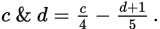If 6 & d = 1.7, what is the value of d?

A. -5
B. -2
C. 0
D. 3
E. 5

2. If each side of an equilateral triangle is 8, what is the approximate measure of the altitude?

A. 1.73
B. 2
C. 3.46
D. 4
E. 6.93

3. For what value of x is f(x) = 6 + (x-2)2 at its minimum?

A. -6
B. -4
C. 0
D. 2
E. 5

4.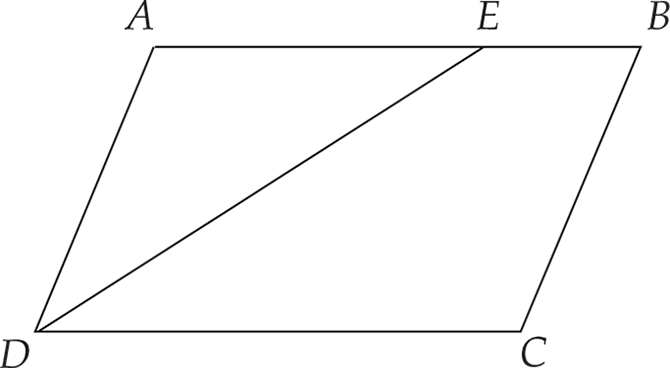Note: Figure not drawn to scale.

In Figure above, If ABCD is a parallelogram, mADE =40°, and mC = 110°, what ismBED?

A. 30°
B. 40°
C. 70°
D. 110°
E. 150°

5. A 3-digitcode is made up of three dIf ferent digits from the set {2, 3, 4, 5, 6}.If 4 is always the first digit in the code, how many 3-digit codes can be formed using each digit only once?

A. 3
B. 5
C. 12
D. 13
E. 20

6.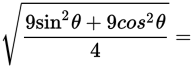A. 1.5
B. 2.25
C. 8.9
D. 23.1
E. 56.12

7. xand Y are independent events. If the probability event xwill occur is 0.2 and the probability event Y will occur is 0.9,what is the probability both x and Y will occur?

A. 18%
B. 20%
C. 50%
D. 72%
E. 90%

8. If x2 - 4x -12 = 0, what is the sum of the two possible values of x?

A. -8
B. -6
C. -2
D. 2
E. 4

9. The number of employees who work at Company A increased by 18% during the year2003. During 2004, the number of employees increased by 26%. What was the approximate percent of increase, to the nearest percent, in employment over the two-year period?

A. 31%
B. 44%
C. 49%
D. 53%
E. 65%

10. If 2 tons of snow fall in 1 minute, how many tons of snow fall in 3 hours?

A. 6
B. 36
C. 360
D. 3,600
E. 4,000

11. A linem with a slope of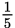passes through the points (-4,1) and (1,y). What is the value of y?

A. -2
B. -1
C. 0
D. 1
E. 2

12. The mean ofa set of 5 numbers is 90. If one of the numbers is removed, the mean of the remaining numbers is 92. What number was removed?

A. 82
B. 84
C. 87
D. 90
E. 92

13. Which statement is true for the set of numbers {6, 7, 11, 6, 8}?

A. mean > mode
B. median > mean
C. mode > median
D. mean = median
E. median = mode

14. A number is randomly selected from the set {6, 7, 8, 8, 8, 10, 10, 11}. What is the probability the number will be less than the mean?

A.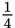B.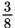C.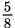D.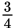E. 1

15. If 3(x + 5) - (x + 2) = 2x- 3x + 4, the n x =

A. -3
B. -1
C. 0
D. 1
E. 3

16. For all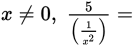A. 5x-2
B. 5x2
C.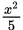D.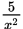E. 25x2

17. (x +y + 3)(x + y + 3) =

A. (x +y)2 + 9
B. x2+y2 + 9
C. x2 +y2 + 6xy + 9
D. (x +y)2 + 6(x + y) + 9
E. (x +y)2 + 9(x + y) + 9

18. The area of square ABCD is three-fourths the area of parallelogramEFGH. the area of parallelogram EFGH is one-third the area of trapezoid IJKL. If square ABCD has an area of 125square feet, what is the area of trapezoid IJKL, in square feet?

A. 75
B. 225
C. 350
D. 500
E. 625

19.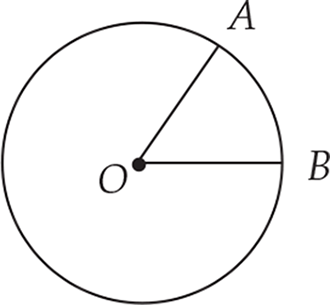Note: Figure not drawn to scale.

The circlein Figure above has center O, minor arc AB = 5π, and mAOB =50°. What is the area of circle O?

A. 25π
B. 324π
C. 576π
D. 1,296π
E. 2,500π

20.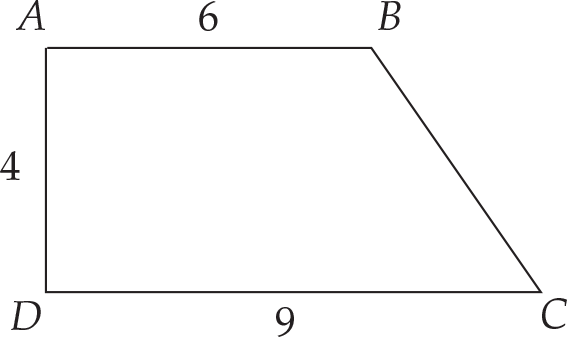Note: Figure not drawn to scale.

In Figure above, ABCD is a trapezoid in which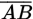is parallel to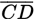,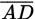is perpendicular to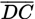, andis perpendicular to. What is the length of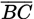?

A. 3
B. 4
C. 5
D. 6
E. 7

﻿